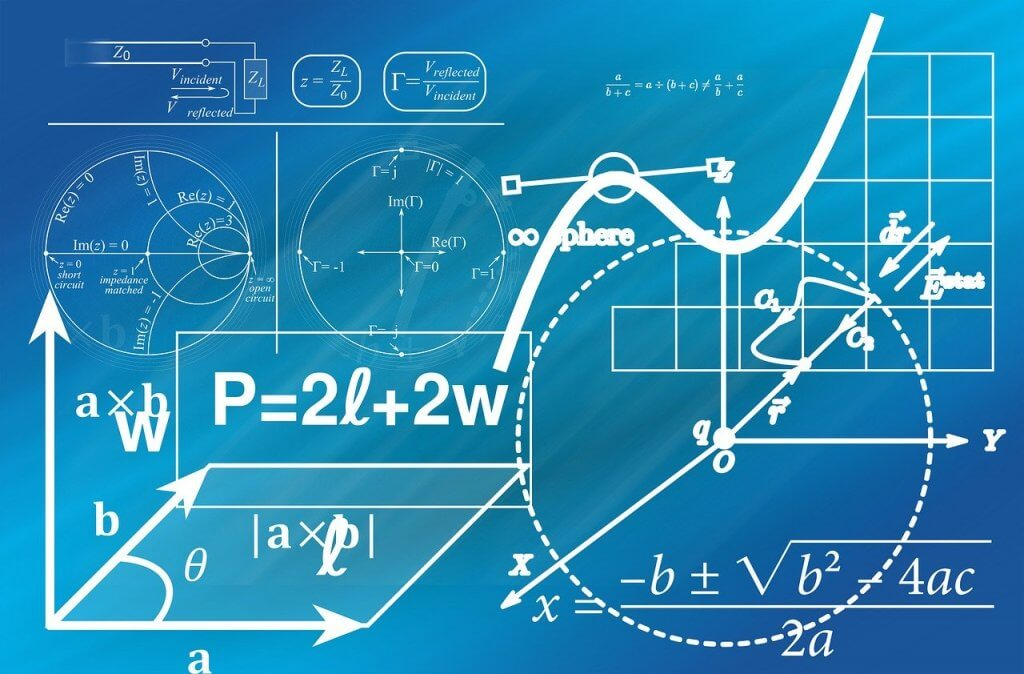# How to do math in a computer

How to do math in a computer? Mathematics is a crucial discipline, which cannot be overlooked, especially in the field of technology. Computers accurately perform mathematical calculations in binary form, which is quite different from what we use: the decimal numbering. Binary typically refers to a numbering system invented by Gottfried Leibniz. In this approach of numbering, a digit has only two possible states or values, i.e. 0 and 1. 0s denote OFF, false or low, and 1s indicate ON, true or high in a transistor. It allows the storage of numbers in a computing device, and therefore calculation becomes possible.

## Using binary digits

A bit is a binary digit. It is the least unit of data in a computing device. The value of a bit is either 0 or 1 when storing informing or executing instructions. For example, decimal numbers 0, 1, 2, 3, 4, 5, 6, 7, 8, 9, 10 are represented in binary form in a computer, as shown below.
0=0, 1=1, 2=10, 3=11, 4=100, 5=101, 6=110, 7=111, 8=1000, 9=1001, 10=1010
Unlike the decimal/denary number system which applies the power of ten (10n), binary uses the power of two (2n) i.e.
Decimal system/ base 10
101 = 10
102= 100
103 = 1,000
106 =1,000,000
Binary system/ base 2
21 = 2
22 = 4
23 = 8
24=16
26 =64
To clearly understand how to do math in a computer, it is essential to note that the calculation of binary starts from right to left. On the left is the most significant bit (MSB), and on the absolute right is the least significant bit (LSB). Starting with “1” from the right, an 8-bit number would be;
128 64 32 16 8 4 2 1
8-bits are equal to 1 byte. When adding in the binary counting system, the bit rules outlined below apply.
0+0=0, 1+0=1, 0+1=1, 1+1=0
Adding all the numbers above equals to 421.
128+64+32+16+8+4+2+1=421
42110 = 1101001012

## Using Boolean logic

Computers operate using Boolean logic. That is a simple method to discover the “true” state of an expression in the binary numbering approach. Boolean operators AND, OR, XOR, and NOT evaluate values to give a true (1) or false (0) outcome. For intricate calculations, the computer processor interlinks several Boolean statements to produce accurate results.

### Conclusion

As illustrated above, mathematics has been used immensely in the advancement of technology. In one way or another, the application of mathematics in our day to day lives has a tremendous positive impact. That is why our math specialists have made it their priority to help students having challenges in math excel. If you want to pay someone to do your math homework, do not hesitate to get in touch with us. Our customer support department is available all the time to offer any form of assistance that you may require.
You do not have to get overwhelmed by complex math problems and assignments, spending sleepless nights trying to beat tight deadlines. At very competitive prices, we ensure your math homework gets completed in time with quality results. Do my college assignment for me? We always guarantee high grades, no matter the complexity level of your math homework. Go ahead and place your order with us today, and you will never regret it.